# Mechanical vibrations - maths tutorial

This tutorial in the series on mechanical vibrations is referred to as the maths tutorial.

This series of tutorials on the subject of mechanical vibrations hinges on solutions to a particular type of differential equation defined as ordinary, second order, linear, homogeneous or non-homogeneous.  The purpose of this tutorial is to explain the general method for these solutions assuming a reasonable knowledge of college level algebra and basic calculus.

• ordinary means that all derivatives of the one dependent variable in the equation are with respect to the same independent variable (i.e. there are no partial derivatives)
• second order means that the highest derivative in the equation is of the second order
• linear means that the exponent of each term containing the dependent variable in the equation is one
• homogeneous equations of this type equal zero;  non-homgeneous equations do not

In addition all terms in the equations considered have constant coefficients.

We use y to denote the dependent variable and x the independent variable.  Thus a solution means finding a function y = f(x) that satisfies the equation in question.

(In the vibration tutorials the dependent and independent variables are usually x (displacement) and t (time) respectively.)

### Solutions to homogeneous equations

We first consider a solution to the homogeneous equation which has the general form: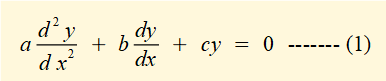In many cases the starting point for solutions to differential equations is to find a function that could be the basis for a solution.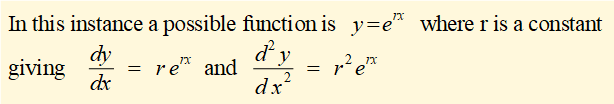Replacing y and its derivatives in (1) above with expressions in erx  gives: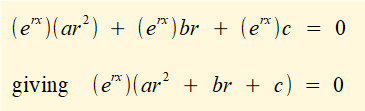Because erx cannot equal zero this equation reduces to:  ar2 + br + c = 0  known as the characteristic equation for the original differential equation.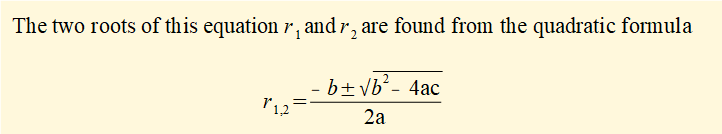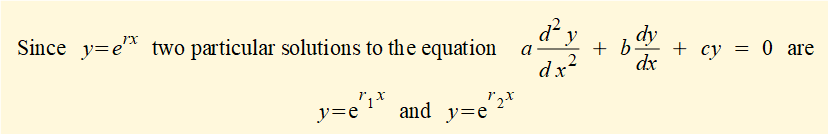It can be shown that any linear combination of these solutions a also a solution.  Hence the general solution can be expressed as: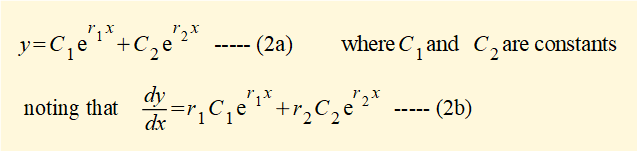A particular solution of the original equation requires valid values of constants C1 and C2.  These are found by setting initial conditions  for y and dy/dx when x = 0 and substituting these values in equations (2a) and (2b) giving two equations with two unknowns (C1 and C2).

At this point we must consider the solutions r1 and r2 of the characteristic equation taking account of the value of the term (b2 - 4ac) in the quadratic formula.

If (b2 - 4ac) > 0 then r1 and r2 are real numbers and the general solution can be obtained directly from equation (2) above.

If (b2 - 4ac) < 0 then r1 and r2 can be expressed as a pair of complex conjugate numbers as follows: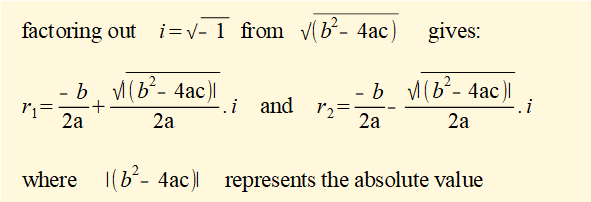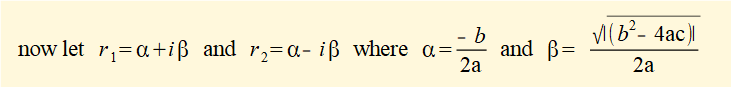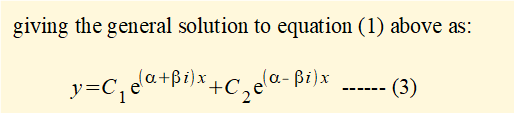Rearranging exponents of e in the RHS of this equation and then using Euler's formula: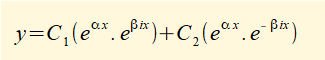leads to a modified general solution to equation (1) above as follows: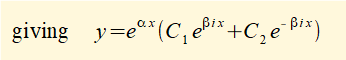Equation (4) has no terms in i making it much easier to apply  than equation (3) above.

Using the identity A.cos(θ) + B.sin(θ) = R.cos(θ - φ)     where R = √(A2 + B2) and  φ = tan-1 (B /A) expresses equation (4) as:

y = R.eαx cos(βx - φ)     where  R = √(C12 + C22)  and  φ = tan-1 (C2 / C1)  --------- (5)

Equation (5) will be found useful since it expresses y in terms only of cosine.

If (b2 - 4ac) = 0 then the characteristic equation has repeated roots, r, but  y = Cerx  is not a complete general solution to equation (1) because two initial conditions, and thus two constants, are required.  However, it can be shown that the following expression (known as reduction of order) does provide a complete general solution for repeated roots:

y = C1erx + C2xerx -------- (5)

In subsequent tutorials we use the general solutions above to solve the homogeneous equations of motion for free vibrating systems.

### Solutions to non-homogeneous equations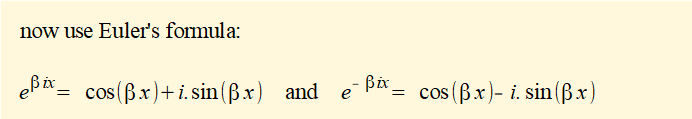The general solution to this form of equation is:

the general solution for the complementary homogeneous equation + the particular solution for the non-homogeneous equation

This is understood better by working through an example for the following non-homogeneous equation:We use a cosine function f(x) = 2cos(3x) in our example because a function of this type arises in non-homogeneous equations of motion for forced vibrating systems.

#### Step 1 - find the general solution to the complementary homogeneous equation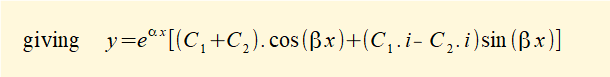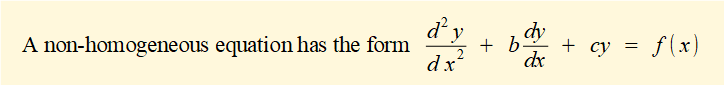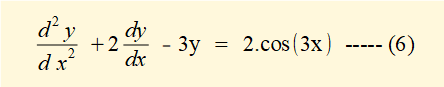#### Step 2 - find the particular solution to the non-homogeneous equation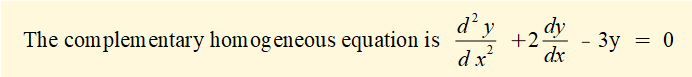Two trig functions whose first and second derivatives are one or other of these functions are an obvious guess.  We propose the following solution: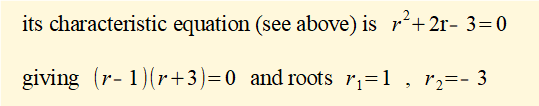If the RHS of equation (6) was an exponential function eax a "guess" would be yp = Aeax and for a polynomial on the RHS a "guess" would be yp = Ax2 +Bx + C

What follows is called the method of undetermined coefficients where we find coefficients A and B by substituting yp, yp/ and yp// in equation (6).

yp/ = - 3.A.sin(3x) + 3.B.cos(3x)

yp// = -9.A.cos(3x) - 9.B.sin(3x)

Substituting in equation (6) gives:

-9.A.cos(3x) - 9.B.sin(3x) -6.A.sin(3x) +6.B.cos(3x)  -3. A.cos(3x) --3.B.sin(3x) = 2.cos(3x)

which gives  ( - 12A + 6.B).cos(3x) + ( - 6.A - 12B).sin(3x)  = 2.cos(3x)

equating coefficients for cos(3x) and sin(3x) on both sides of the equation gives:

( - 12A + 6.B) = 2    and    ( - 6.A - 12B) = 0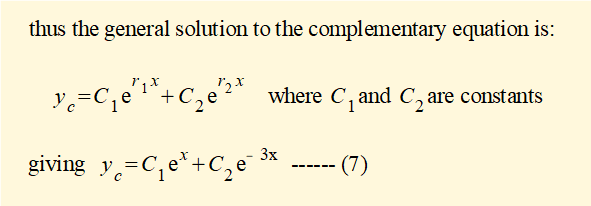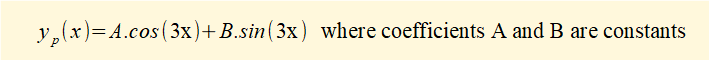#### Step 3 - combine solutions

The general solution yg to the non-homogeneous equation (6) is a combination of equation (7),  the solution yc to the complementary homogeneous equation, and equation (8), the particular solution yp to equation (6) which gives: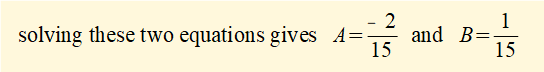I welcome feedback at:

### Tutorials - mechanical vibrations

###### Forced vibrations with damping

Alistair's tutorials 2021